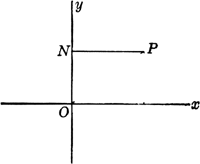# abscissa

(redirected from abscissas)
Also found in: Dictionary, Thesaurus, Medical.
Related to abscissas: Coordinate axes, ordinates

## Cartesian coordinates

Cartesian coordinates (kärtēˈzhən) [for René Descartes], system for representing the relative positions of points in a plane or in space. In a plane, the point P is specified by the pair of numbers (x,y) representing the distances of the point from two intersecting straight lines, referred to as the x-axis and the y-axis. The point of intersection of these axes, which are called the coordinate axes, is known as the origin. In rectangular coordinates, the type most often used, the axes are taken to be perpendicular, with the x-axis horizontal and the y-axis vertical, so that the x-coordinate, or abscissa, of P is measured along the horizontal perpendicular from P to the y-axis (i.e., parallel to the x-axis) and the y-coordinate, or ordinate, is measured along the vertical perpendicular from P to the x-axis (parallel to the y-axis). In oblique coordinates the axes are not perpendicular; the abscissa of P is measured along a parallel to the x-axis, and the ordinate is measured along a parallel to the y-axis, but neither of these parallels is perpendicular to the other coordinate axis as in rectangular coordinates. Similarly, a point in space may be specified by the triple of numbers (x,y,z) representing the distances from three planes determined by three intersecting straight lines not all in the same plane; i.e., the x-coordinate represents the distance from the yz-plane measured along a parallel to the x-axis, the y-coordinate represents the distance from the xz-plane measured along a parallel to the y-axis, and the z-coordinate represents the distance from the xy-plane measured along a parallel to the z-axis (the axes are usually taken to be mutually perpendicular). Analogous systems may be defined for describing points in abstract spaces of four or more dimensions. Many of the curves studied in classical geometry can be described as the set of points (x,y) that satisfy some equation f(x,y)=0. In this way certain questions in geometry can be transformed into questions about numbers and resolved by means of analytic geometry.

## abscissa

[ab′sis·ə]
(mathematics)
One of the coordinates of a two-dimensional coordinate system, usually the horizontal coordinate, denoted by x.
McGraw-Hill Dictionary of Scientific & Technical Terms, 6E, Copyright © 2003 by The McGraw-Hill Companies, Inc.

## abscissaabscissa: P, any point; NP, abscissa
In the plane Cartesian coordinate system, the horizontal coordinate of a point on a plane; the x-coordinate, obtained by measuring the distance from the point to the y-axis along a line parallel to the x-axis.
McGraw-Hill Dictionary of Architecture and Construction. Copyright © 2003 by McGraw-Hill Companies, Inc.

## abscissa

the horizontal or x-coordinate of a point in a two-dimensional system of Cartesian coordinates. It is the distance from the y-axis measured parallel to the x-axis
Collins Discovery Encyclopedia, 1st edition © HarperCollins Publishers 2005

## abscissa

(mathematics)
The horizontal or x coordinate on an (x, y) graph; the input of a function against which the output is plotted.

The vertical or y coordinate is the "ordinate".

See Cartesian coordinates.
References in periodicals archive ?
These methods are specified by the abscissa vector c = [[[c.sub.1], ..., [c.sub.s].sup.T] [member of] [R.sup.s], four coefficient matrices
Try to describe this behavior in terms of the corresponding abscissas of the iterates, instead of referring to the points where the spiral changes direction.
The lines intersect the straight hyperbolas at two points, and also intersect the real axis and the abscissa axis where they intercept different lengths.
This process is repeated for all abscissas obtained in step 2.
The same abscissas [x.sub.s] can be employed to generate one more quadrature.
Finally, the reconstruction of the zone between the abscissas 18.5 and 21.5 m (from the beginning of the line, corresponding to the zone of the church of San Rocco) is considered for the second scan line (see Figure 10).
2 and 3 (for Kentucky bluegrass and perennial ryegrass, respectively) where the ordinates are the IAS-1 genotype parameters ([[lambda].sup.0.5] [[??].sub.g]) and the abscissas are the means for genotypes (averaged over environments).
Let f be coordinates of a plane and g be abscissas of points of that plane, then h = f - g are ordinates of points of the plane.
+ [a.sub.1]t + [a.sub.0] is a Hurwitz polynomial (n [greater than or equal to] 2) and [[sigma].sub.p] and [[sigma].sub.p'] are the abscissas of stability of p and p' = dp/dt, respectively, then [[sigma].sub.p'] [less than or equal to] [[sigma].sub.p].
The axis of abscissas shows the current values of [S.sub.i] in doles of the initially value.
em que: [Y.sub.t] e o peso corporal, em kg; t e o tempo, em dias; [a.sub.1] e [a.sub.2] sao os pesos maximos (estabilizacao do crescimento), respectivamente para as fases 1 e 2; [k.sub.1] e [k.sub.2] sao as taxas de maturidade (velocidade de crescimento ou precocidade), respectivamente para as fases 1 e 2; [d.sub.1] e [d.sub.2] sao as abscissas do ponto de inflexao da curva (nao apresenta significado biologico), respectivamente para as fases 1 e 2; e t e o residuo no tempo t; [e.sub.t-1] e o residuo no tempo t-1; [e.sub.t-2] e o residuo no tempo t-2, e os parametros [[phi].sub.1] e [[phi].sub.2] correspondem, respectivamente, aos parametros de auto-regressao residual de primeira e segunda ordem.

Site: Follow: Share:
Open / Close